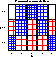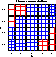##Sieve DiagramsA sieve diagram shows the frequencies in a two-way contingency table in relation to expected frequencies under independence, and highlights the pattern of association between the row and column variables. The sieve diagram was proposed by Riedwyl and Schüpbach in a technical report in 1983 and later called a parquet diagram (Riedwyl and Schüpbach, 1994).

### Constructing the sieve diagram

In this display:

• A unit square is divided into rectangles, one for each cell in the contingency table.
• The height of each rectangle in row i is proportional to the marginal frequency in that row ( f i+ ); the width of each rectangle in column j is proportional to the marginal frequency in that column ( f +j ).
• Hence, the area of each rectangle is proportional to the expected frequency,
e ij = (f i+ f +j ) / f ++
under the hypothesis that the row and column variables are independent.
• The observed frequency in each cell is shown by the number of squares drawn in each rectangle.
• Hence, the difference between observed and expected frequency appears as the density of shading, using color to indicate whether the deviation from independence is positive (blue) or negative (red).

### Examples, Demo, Software

Examples
This section from my short course, Categorical Data Analysis with Graphics shows several examples.
Form-based Demo